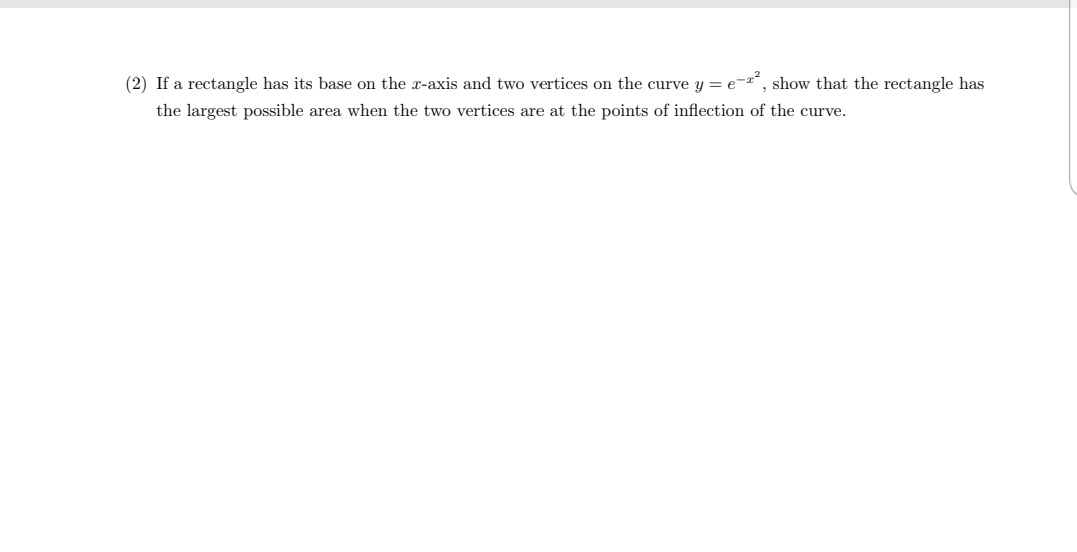# If a rectangle has its base on the r-axis and two vertices on the curve y = e-", show that the rectangle has the largest possible area when the two vertices are at the points of inflection of the curve.

Question

If a rectangle has its base on the x-axis and two vertices on the curve y=e^-x^2, show that the rectangle has the largest possible area when the two vertices are at the points of inflection of the curve.help_outlineImage TranscriptioncloseIf a rectangle has its base on the r-axis and two vertices on the curve y = e-", show that the rectangle has the largest possible area when the two vertices are at the points of inflection of the curve. fullscreen

### Want to see this answer and more?

Experts are waiting 24/7 to provide step-by-step solutions in as fast as 30 minutes!*

*Response times may vary by subject and question complexity. Median response time is 34 minutes for paid subscribers and may be longer for promotional offers.
Tagged in
Math
Calculus

### Applications of Derivative